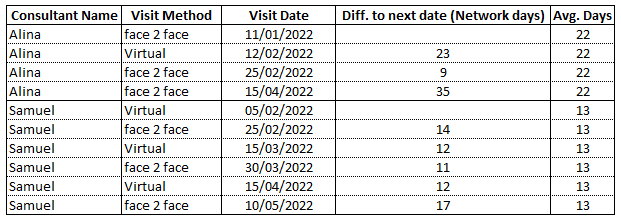# Calculate network days from date with single column

Dear User,

I would like to have some support regarding the calculation of network days from a single column of date field and then I need to calculate the average of network days.
Average of network days is relatively easier as I need to use ‘groupby’ node. I would like to understand how can I calculate network days from single column per consultant as shown in image.datetime difference node can give you the difference
br

You can use a loop or mark the instance a new patient appears (this is the method I used):

Network_Days.knar.knwf (24.1 KB)

2 Likes

@ngumpena

And here the option with a loop: calculate_networkdays.knwf (36.9 KB)

gr. Hans

1 Like

Thank you very much for the reply.

Network days calculation means to consider only Monday to Friday in the calculation.

This is where mostly I’m looking for support. We need to get a match with the number in the image.

The numbers you provided seem off by 1 when I did a manual check. If you require the numbers to be off by 1, i.e. to not take into account the day seen, you can add the Math Formula node (\$patient_network_days\$ - 1) to this new workflow:

Network_Days(2).knar.knwf (40.9 KB)

Can I also ask what is the purpose of calculating network days given you have dates that do happen on the weekends?